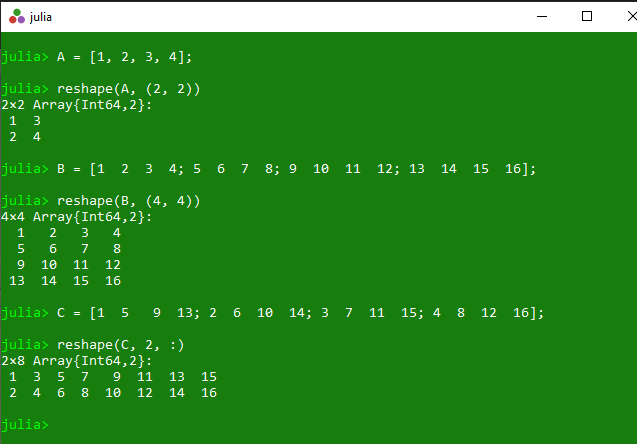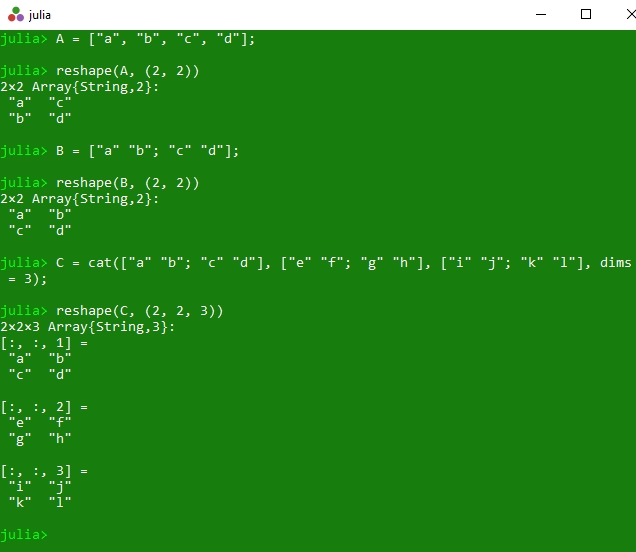# Reshaping array dimensions in Julia | Array reshape() Method

• Last Updated : 23 Mar, 2020

The `reshape()` is an inbuilt function in julia which is used to return an array with the same data as the specified array, but with different specified dimension sizes.

Syntax:
reshape(A, dims)

Parameters:

• A: Specified array.
• dims: Specified dimension.

Returns: It returns an array with the same data as the specified array, but with different specified dimension sizes.

Example 1:

 `# Julia program to illustrate ``# the use of Array reshape() method`` ` `# Getting an array with the same data``# as the specified 1D array, but with``# (2 * 2) dimension sizes.``A ``=` `[``1``, ``2``, ``3``, ``4``];``println(reshape(A, (``2``, ``2``)))`` ` `# Getting an array with the same data``# as the specified 2D array, but with``# (4 * 4) dimension sizes.``B ``=` `[``1`  `2`  `3`  `4``; ``5`  `6`  `7`  `8``; ``9`  `10`  `11`  `12``; ``13`  `14`  `15`  `16``];``println(reshape(B, (``4``, ``4``)))`` ` `# Getting an array with the same data``# as the specified 2D array, but with``# (2 * 8) dimension sizes.``C ``=` `[``1`  `5`   `9`  `13``; ``2`  `6`  `10`  `14``; ``3`  `7`  `11`  `15``; ``4`  `8`  `12`  `16``];``println(reshape(C, ``2``, :))`

Output:Example 2:

 `# Julia program to illustrate ``# the use of Array reshape() method`` ` `# Getting an array with the same data``# as the specified 1D array, but with``# (2 * 2) dimension sizes.``A ``=` `[``"a"``, ``"b"``, ``"c"``, ``"d"``];``println(reshape(A, (``2``, ``2``)))`` ` `# Getting an array with the same data``# as the specified 2D array, but with``# (2 * 2) dimension sizes.``B ``=` `[``"a"` `"b"``; ``"c"` `"d"``];``println(reshape(B, (``2``, ``2``)))`` ` `# Getting an array with the same data``# as the specified 3D array, but with``# (2 * 2*3) dimension sizes.``C ``=` `cat([``"a"` `"b"``; ``"c"` `"d"``], [``"e"` `"f"``; ``"g"` `"h"``], [``"i"` `"j"``; ``"k"` `"l"``], dims ``=` `3``);``println(reshape(C, (``2``, ``2``, ``3``)))`

Output:My Personal Notes arrow_drop_up